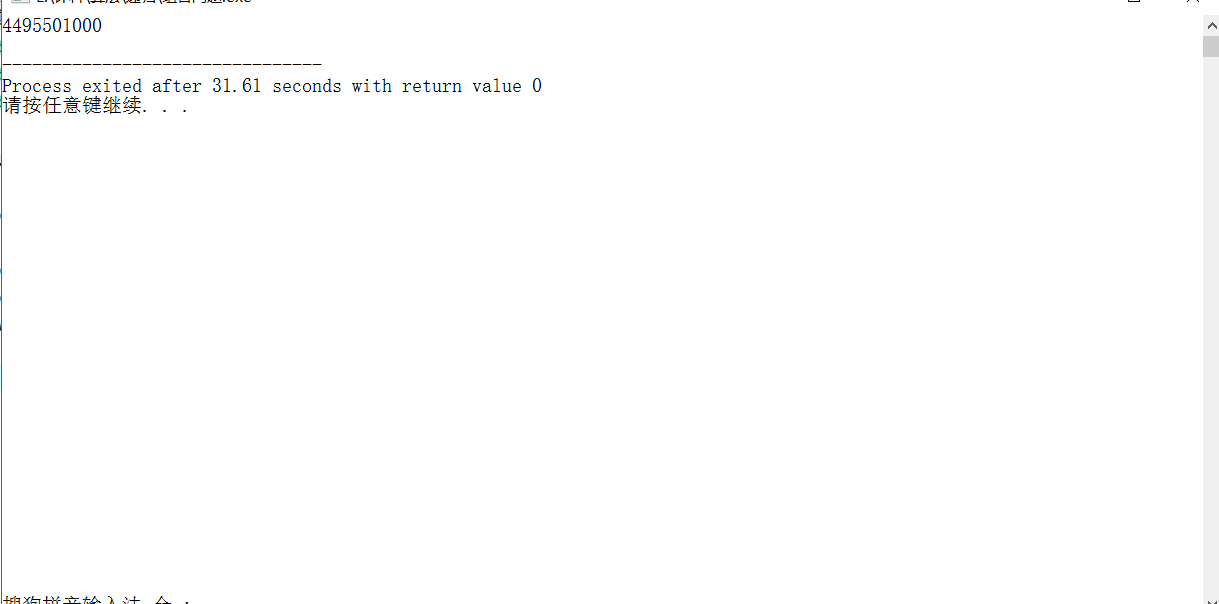• <noscript id="e0iig"><kbd id="e0iig"></kbd></noscript>
• <option id="e0iig"></option>
• <noscript id="e0iig"><source id="e0iig"></source></noscript>

目錄

《一》構造遞歸

《二》遞歸的調用

構造遞歸代碼

《三》遞歸和回溯

更多代碼

《四》遞歸的局限性

小結

## 《一》構造遞歸

抓住兩個要點：“相似性”和“出口

沒有相似性，主動構造

不相似的原因很有可能是缺少參數

類似數學公公式上的遞推

## 《二》遞歸的調用

1. 被調函數恰為主調函數
2. 調用次序不同
• 遞歸的調用離不開棧，棧的作用是后進先出

• 棧會保存斷點的信息，待執行完被調函數之后返回主調函數從斷點處繼續執行

3. 注意返回次序（后進先出）
4. 形參并非同一變量
1. 雖然傳遞的都是同一個參數，但他們本質上是不同的
2. 比如：山上有個廟廟里有個老和尚這樣的循環，老和尚所在的山是山1，他所講的山是山2

### 構造遞歸代碼

``````# include<cstdio>

#if 0
構造遞歸：
先考慮共性函數，再對終止條件做限制

共性函數的設計不同，其遞歸函數的結構也不同
# endif

//從begin打印到end 先打印begin(1)
void f(int begin,int end)
{
/*
當發現遞歸沒有共通性時，可以考慮增加參數
*/
if(begin > end)
return ;
printf("%d ",begin);
f(begin+1,end);
}

//打印1~n  先打印9（從大到小打印）
void f2(int n){
if(n > 1)
f2(n-1);
printf("%d ",n);
}

int main()
{
int n = 9;
f(1,n);//打印0-n
printf("\n");
f2(n);

return 0;
} ``````
`````` /*數組求和*/
# include<cstdio>

/*三種思路
*(1)a[begin] + (a[begin+1]+...+a[end])
*(2)(a[begin] + a[end-1]) + ... a[end]
*(3)折半相加
*/

int length(int a[])
{
int len = 0;
for(int i = 0;a[i];++i)
len++;
return len;
}

/*兩種基本遞歸求和*/
int f1(int a[],int begin)
{
if(begin == length(a))
return 0;
int x = f1(a,begin+1);
return x + a[begin];
}

int f2(int a[],int end)
{
if(end < 0)
return 0;
int x = f2(a,end-1);
return x + a[end];
}

/*折半相加*/
int f3(int a[],int begin,int end)
{
int mid = (begin+end)/2;
if(mid == end)
return a[end];
if(begin == mid)
return a[mid];

return f3(a,begin,mid) + f3(a,mid,end);
}

int main()
{
int a[] = {2,5,8,9,6,3,1,4,7};

int begin = 0;
//int sum1 = f1(a,begin);
//int sum2 = f2(a,length(a));
int sum3 = f3(a,begin,length(a));
printf("%d",sum3);

return 0;
} ``````
``````# include<iostream>
# include<string>

using namespace std;

/*判斷兩個串是否相等*/
bool f(string& s1,string& s2){
if(s1.length() != s2.length())
return false;
if(s1.length() == 0)
return true;

if(s1 != s2)
return false;
string ss1 = s1.substr(1,s1.length());
string ss2 = s2.substr(1,s2.length());
return f(ss1,ss2);
}

int main()
{
string s1,s2;

cout << "輸入串1:" << endl;
getline(cin,s1);
cout << "輸入串2:" << endl;
getline(cin,s2);

if(f(s1,s2))
cout << "相等!" << endl;
else
cout << "不相等!" << endl;

return 0;
}``````

## 《三》遞歸和回溯

注意:

每一次調用都要降低計算規模

找到相似性

定義出口

注意出口的順序

注意回溯

回溯的作用就是保持單一變量

回溯代碼：

``````# include<iostream>
# include<algorithm>
# include<string>

using namespace std;

/*全排列遞歸算法 */
#if 0
相似性:
字符串中的每一個字符都可以做第一位（遍歷字符串中的每一個字符），確定當前一位，之后依次確定
出口:
字符串中的每一個字符都放到過第一位
#endif
//k:記錄當前交換位置
void f(string &s,int k){
if(k == s.length()){
for(int i = 0;i < s.length();++i)
cout << s[i] << " ";

cout << endl;
}

//循環遍歷時，需要注意回溯 回歸到原狀態，來確保只有單一變量
for(int i = k;i < s.length();++i)
{
int t = s[k]; s[k] = s[i]; s[i] = t;//交換
f(s,k+1);
t = s[k]; s[k] = s[i]; s[i] = t;//回溯
}
}

int main()
{
string str = "abc";

f(str,0);

return 0;
}
``````
``````# include<iostream>
# include<algorithm>
# include<string>

using namespace std;

/*求最大公共子序列個數*/
//遞歸

/*
相似性:
s1的第一個字符和s2的字符
{
相等:截取兩個串的第一個的串比較
不相等:分別截取s1和s2的首字符求兩個最大公共子串的最大值
}

出口:
任一個子串的長度為0
*/
int f(string &s1,string &s2){

if(s1.length() == 0 || s2.length() == 0)
return 0;

string ss1 = s1.substr(1,s1.length());
string ss2 = s2.substr(1,s2.length());
if(s1.at(0) == s2.at(0))
return f(ss1,ss2)+1;
else
return max(f(ss1,s2),f(s1,ss2));
}

int main()
{
string s1,s2;

getline(cin,s1);
getline(cin,s2);

cout << f(s1,s2);

return 0;
}
``````

### 更多代碼

``````# include<iostream>
# include<algorithm>

using namespace std;

/*遞歸解法
相似性：
思路就是從n個球中選一個特殊的，要么選【f(n-1,m-1)】，要么不選【f(n-1,m)】
出口:
n < m     --> 0
n == m    --> 1
m == 0    -->1
*/
long long f(int n,int m){
if(n < m)
return 0;
if(n == m)
return 1;
if(m == 0)
return 1;

return f(n-1,m-1) + f(n-1,m);
}

/*優化算法*/
long long f1(int n,int m){

}

int main()
{
cout << f(3000,3) << endl;

return 0;
}````````````# include<iostream>
# include<algorithm>
# include<string>

using namespace std;

/*字符串反轉遞歸實現*/
string reverse(string &s){
if(s.length() < 2) return s;
string ss = s.substr(1,s.length());
return reverse(ss) + s.at(0);
}

int main()
{
string s;

cin >> s;
cout << reverse(s) << endl;

return 0;
}
``````
``````# include<iostream>
# include<algorithm>

using namespace std;

#if 0
楊輝三角可以轉化成下三角矩陣的形式
1                 1
1   1               1  1
1  2  1              1  2  1
1  3  3  1       ===> 1  3  3  1
1  4  6  4  1          1  4  6  4  1
1  5 10 10  5 1	       1  5 10 10  5  1
# endif

//輸出楊輝三角的第m層第n個元素的系數
int f(int m,int n){
if(m == 0 || n == 0) return 1;
if(n == m) return 1;
return f(m-1,n) + f(m-1,n-1);
}

int main()
{
int m;

cout << "輸入楊輝三角的層數:";
cin >> m;
m -= 1;
for(int i = 0;i <= m;++i)
cout << f(m,i) << " ";

return 0;
}
``````
``````# include<iostream>
# include<algorithm>

using namespace std;

int n = 5;

/*計算錯誤和*/
//err_sum:錯誤和
//a[]:數組
//k:當前項
//cur_sum:當前和
//b[]:是否取當前項
void f(int err_sum,int a[],int k,int cur_sum,bool b[]){

if(cur_sum > err_sum)	return ;
if(cur_sum == err_sum){
for(int i = 0;i < n;++i)
if(b[i])
cout << a[i] << " ";
cout << endl;
return ;
}
if(k >= n)	return ;

//分兩種情況:是否取當前項
b[k] = false;
f(err_sum,a,k+1,cur_sum,b);

b[k] = true;
cur_sum += a[k];
f(err_sum,a,k+1,cur_sum,b);
b[k] = false;
}

int main()
{
int err_sum = 6;

int a[] = {3,2,4,3,1};
bool *b = new bool[n];

for(int i = 0;i < n;++i)
b[i] = false;
f(err_sum,a,0,0,b);

return 0;
}
``````

## 《四》遞歸的局限性

一、函數調用需要使用內存中的棧來保存函數的數據以及訪問鏈和控制鏈，如果數據是必須的，那么訪問鏈和控制鏈等所占的內存則是額外的。
二、使用遞歸，會進行很深層次的調用函數，所以需要調用很多函數，需要建立許多的訪問鏈和控制鏈，占用大量內存，而且調用時傳遞參數，申請空間，返回時恢復現場，都有時間的花銷，所以遞歸效率低。
三、內存的棧當作一個容器，每次遞歸，函數都往容器中添加容量，當添到一定層次，容器滿了，就溢出了，如果用遞歸，如果忘記了遞歸截至條件，你就可能進入了無窮遞歸，無窮遞歸必然會溢出，所以，不提倡遞歸。

### 小結

遞歸算法一般都是提供一種思路，對于規模較小可以解決，但遠遠不能滿足正常規模的輸入（如組合問題中，才3000就需要30多秒，這已經算掛了），所以常常需要優化算法。

部分優化算法：

### 遞歸

遞歸的定義 1.遞歸有兩種方式：直接遞歸和間接遞歸，通俗的理解為方法體里面調用方法本身。 直接遞歸：直接調用自己稱為直接遞歸 間接遞歸：間接調用自己稱為間接遞歸 遞歸的語句寫起來相當簡單，但是，理解起來有些困難，一般的循環語句，我們都可以將它寫成遞歸的方式，減少代碼的冗余度。 3.遞歸的模型 遞歸的步驟我們可以分為3步。 ① 找重復————&mdas...

### 遞歸

遞歸 回顧 遞歸規則： 當程序執行到一個方法時，就會開辟一個獨立的空間(棧) 每個空間的數據(局部變量)，是獨立的 遞歸需要遵守的重要規則 執行一個方法時，就創建一個新的受保護的獨立空間(棧空間) 方法的局部變量是獨立的，不會相互影響。 如果方法中使用的是引用類型變量(比如數組)，就會共享該引用類型的數據. 遞歸必須向退出遞歸的條件逼近，否則就是無限遞歸,出現StackOverflowError，...

### 遞歸

定義：在一個函數中再次調用該函數自身的行為叫做遞歸,這樣的函數被稱作遞歸函數。 基礎應用：有明顯遞歸式 例如，我們想要編寫一個計算階乘的函數int fact(int n)，當然，用循環來實現也是可以的。但是根據階 乘的遞推式n! =n*(n-1)!,我們可以寫成如下形式:  在編寫-一個遞歸函數時，函數的停止條件是必須存在的。在剛剛的例子中，當n=0時fact并不是繼續調用自身，而是直接...

### 遞歸

遞歸調用機制原理圖： 迷宮問題： 八皇后問題： 在8×8棋盤上，有兩個棋子，不能在同一行，同一列，同一斜線。有多少種擺法？ 可以用一維數組表示上圖擺法：arr = {0,4,7,5,2,6,1,3} arr下標表示第幾行 arr的值表示列數...

### 遞歸

簡單的說: 遞歸就是方法自己調用自己,每次調用時傳入不同的變量.遞歸有助于編程者解決復雜的問題,同時可以讓代碼變得簡潔。 遞歸需要遵守的重要規則 執行一個方法時，就創建一個新的受保護的獨立空間(棧空間) 方法的局部變量是獨立的，不會相互影響, 比如n變量 如果方法中使用的是引用類型變量(比如數組)，就會共享該引用類型的數據. 遞歸必須向退出遞歸的條件逼近，否則就是無限遞歸,出現StackOverf...

### 遞歸

遞歸是一種方法（函數）調用自己的編程技術。 知道遞歸這兩個字的時候是在大學生活時代的C語言的課堂上，當時老師提到了遞歸，說這是一種一個函數自己調用自己的方法，然后就非常感興趣，感覺非常的神奇，但是當自己實際用到的時候，卻怎么都理解不了他的好處，它是怎么實現之類的，就不像 if-else 語句這種，學的時候就可以在大腦中刻畫一個流程圖出來，然后可以很快的理解，所以當時出于對知識的敬畏，就沒有在深入研...

### 遞歸

遞歸 1.如何理解“遞歸” 2.遞歸需要滿足的三個條件 3.如何編寫遞歸代碼 4.編寫遞歸代碼時的常見問題：堆棧溢出、重復計算 1 堆棧溢出 2 重復計算 5 怎么將遞歸代碼改寫為非遞歸代碼？ 1 例子1 2 例子2 6 如何用三行代碼找到“最終推薦人”？ 7 總結 8 思考題 1.如何理解“遞歸” 遞歸是一種應用非常廣泛的算...

### 遞歸

文章目錄 遞歸 1. 遞歸的概念 2. 迷宮問題 3. 八皇后問題（回溯算法） 4. 漢諾塔問題 遞歸 1. 遞歸的概念 簡單的說：遞歸就是方法自己調用自己，每次調用時傳入不同的變量.遞歸有助于編程者解決復雜的問題，同時可以讓代碼變得簡潔。 遞歸調用機制 1 打印問題 2 階乘問題 使用圖解的方式說明遞歸的調用機制 遞歸能解決什么樣的問題 1）各種數學問題如：8皇后問題，漢諾塔，階乘問題，迷宮問題...

### 遞歸

遞歸 遞歸：方法自己調用自己 遞歸的分類： 內存溢出代碼演示 遞歸 使用遞歸求n 的階乘 使用遞歸遍歷文件夾目錄 遞歸：方法自己調用自己 遞歸的分類： 注意： 遞歸一定要有條件限定，保證遞歸能夠停下來，否則會發生棧內存溢出。 遞歸次數不能太多，否則也會發生棧內存溢出。 構造方法禁止遞歸。 內存溢出代碼演示 沒有條件限定，遞歸會發生異常：i方法在棧內一直調用i方法，導致棧內有無數個i方法，最后太多超...

### 遞歸

...

精品国产乱码久久久久久蜜桃不卡